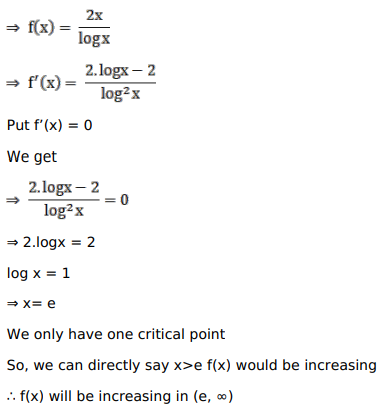# Solve this following

Question:

Mark $(\sqrt{ })$ against the correct answer in the following:.

$f(x)=\frac{2 x}{\log x}$ is increasing in

A. $(0,1)$

B. $(1, \mathrm{e})$

C. $(e, \infty)$

D. $(-\infty$, e $)$

Solution: